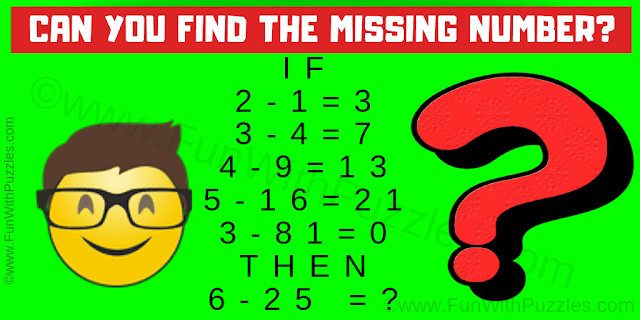This #Maths #Reasoning Question will make you think outside the box. In this Maths Logic Puzzle, you are shown some number equation. These are the logical equations for which you need to decipher the hidden logic and then find the value of the missing number which replaces the question mark.
This is a very interesting logic math puzzle which will make you think outside the box. First four logical equations in this puzzle lead to the very easy logic. However 5th statement will make you think outside the box. Can you find this hidden logic and then solve the last logical equation?Can you find the missing number?
Answer of this "Maths Reasoning Question", can be viewed by clicking on the answer button.